# How to use IF function in excel

Updated on Fri Jan 01 2021

SHARE

In this tutorial you can learn how to use the Microsoft Excel's IF Function.
Function Arguments of Excel's IF Function are given below.

1. First argument is the condition ( A comparison operator like equal, greater than etc. or a worksheet function that return TRUE or FALSE).

2. Second argument is what to do if the condition is true – this can be any Excel formula like “=D5″,”=SUM(A2:A10)” or value like “0”,”25″ etc.

3. Third argument is what to do if the condition is FALSE – this can also be any Excel formula like “=D5”,”=SUM(A2:A10) or value like “0”,”25″ etc. This third argument is optional which means it can be omitted if it not needed.

Use the IF function in Excel as described in the steps given below.

1. Step 01

Type in “=IF(” in where you want to have the return value or the final output.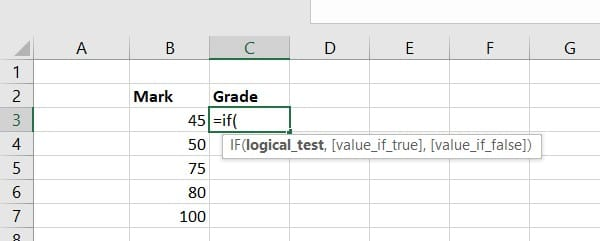2. Step 02

Type the condition that returns TRUE or FALSE as the return value. Ex:- C5>10, A10=0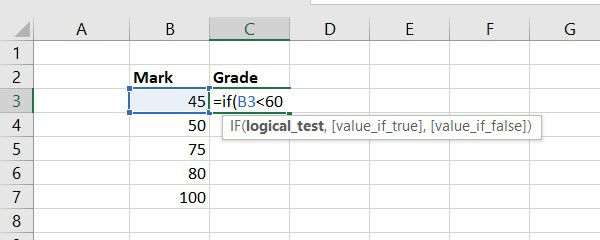3. Step 03

Type the second argument of the if function followed by a comma (,). This could be any excel formula, value or text to output as the result if the condition become TRUE.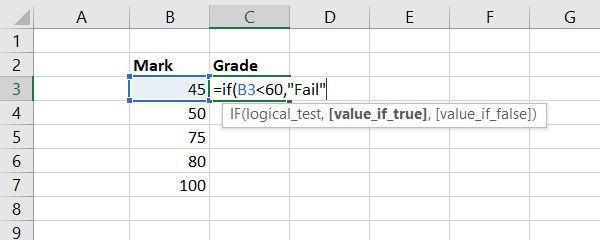4. Step 04

Type the third argument of the if function followed by a comma (,). This could be any excel formula, value or text to output as the result if the condition become FALSE.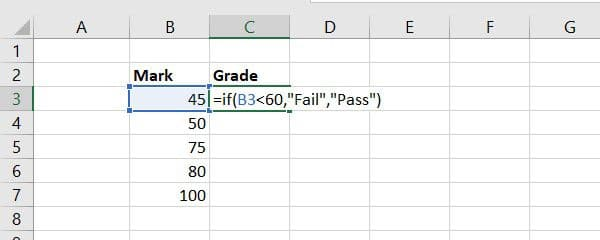5. Step 05

Close the parentheses and hit “Enter”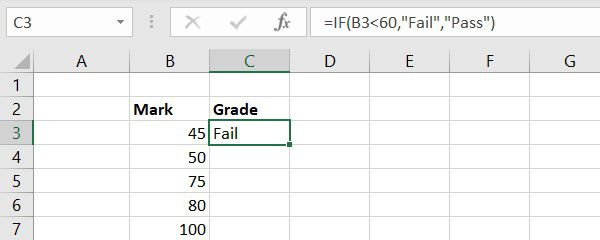##### Tools
• Microsoft Excel

Hope you understood how to use IF Function clrealy.If you have encounter with any issue using if function, or you want to have more complex case, feel free let us hear about it in the comment section below.